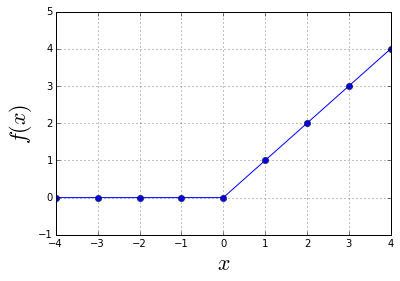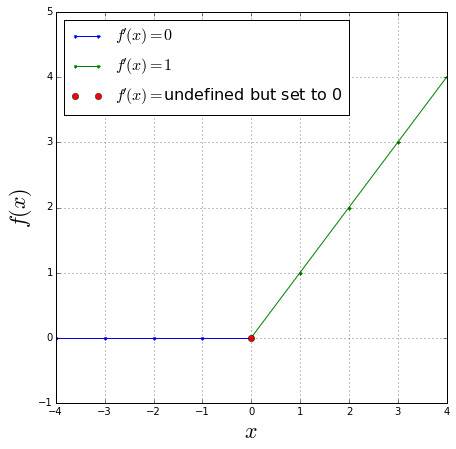var myUrl = 'http://kawahara.ca/what-is-the-derivative-of-relu/'; if((window.top.location.href !== myUrl) || (window.top.location.ref !== 'http://kawahara.ca) || (window.top.location.ref !== 'http://www.kawahara.ca)) { window.top.location.href = myUrl; } 

Short Summary

The rectified linear unit (ReLU) is defined as $f(x)=\text{max}(0,x)$. The derivative of ReLU is:

\begin{equation} f'(x)= \begin{cases} 1, & \text{if}\ x>0 \\ 0, & \text{otherwise} \end{cases} \end{equation}

/end short summary

If you want a more complete explanation, then let's read on!

In neural networks, a now commonly used activation function is the rectified linear unit, or as commonly abbreviated, ReLU. The ReLU is defined as,

\begin{equation} f(x) = \text{max}(0,x) \end{equation}

What does this function do? Basically, it sets anything less than or equal to 0 (negative numbers) to be 0. And keeps all the same values for any values > 0. Let's do some examples and plot this out to make sure we are clear.

In :
# Import needed libraries and other python stuff here.
# Show figures directly in the notebook.
%matplotlib inline
import matplotlib.pyplot as plt # For plotting.
import numpy as np # To create matrices.


ReLU

In :
# Here we define the ReLU function.
def f(x):
"""ReLU returns 1 if x>0, else 0."""
return np.maximum(0,x)

In :
# If we give ReLU a positive number, it returns the same positive number.
print f(1)
print f(3)

1
3

In :
# If we give ReLU a negative number, it returns 0.
print f(-1)
print f(-3)

0
0

In :
# If we give ReLU a value of 0, it also returns 0.
f(0)

Out:
0

Derivative of ReLU

Now just looking at the equation $f(x) = \text{max}(0,x)$, it was not clear to me what the derivative is, i.e. what is the derivative of the max() function?

However, the derivative becomes clearer if we graph things out.

Let's start by creating a range of x values, starting from -3 to +3, and increment by 0.5

In :
X = np.arange(-4,5,1)
print X

[-4 -3 -2 -1  0  1  2  3  4]


Then, we compute the ReLU for all the X values.

In :
Y = f(X)
# All negative values are 0.
print Y

[0 0 0 0 0 1 2 3 4]


We can plot $X$ along the x-axis, and $f(X)$ along the y-axis to see how how the ReLU function looks.

In :
plt.plot(X,Y,'o-')
plt.ylim(-1,5); plt.grid(); plt.xlabel('$x$', fontsize=22); plt.ylabel('$f(x)$', fontsize=22)

Out:
<matplotlib.text.Text at 0x3177c50>A "derivative" is just the slope of the graph at certain point. So what is the slope of the graph at the point $x=2$?

We can visually look at the segment where x=2 and see that the slope is 1. In fact, this holds everywhere >0. The slope is 1.

What is the slope of the graph when x=-2? Visually, we see that there is no slope (change in Y), so the slope is 0. In fact, for all negative numbers, the slope is 0.

Let's graph the same plot again, but this time, plot all negative x values in blue, and all positive x values in green.

In :
plt.figure(figsize=(7,7))

X_neg = np.arange(-4,1,1) # Negative numbers.
plt.plot(X_neg,f(X_neg),'.-', label='$f\'(x) =0$'); # Plot negative x, f(x)

X_pos = np.arange(0,5,1) # Positive numbers
plt.plot(X_pos, f(X_pos), '.-g',label='$f\'(x)=1$') # Plot positive x, f(x)

plt.plot(0,f(0),'or',label='$f \'(x)=$undefined but set to 0') # At 0.

plt.ylim(-1,5); plt.grid(); plt.xlabel('$x$', fontsize=22); plt.ylabel('$f(x)$', fontsize=22) # Make plot look nice.
plt.legend(loc='best', fontsize=16)

Out:
<matplotlib.legend.Legend at 0x33d7550>Now what about x=0? Technically this is undefined. When x=0, there are many possible lines (slopes) we could fit through it. So what do we do here?

Basically we just choose a slope to use when x=0. A common choice is when x=0, the derivative will be 0. It could be some other value, but most implementations use this (this has a nice property that it encourages many values to be 0 i.e., sparsity in the feature map).

Alright there you go. We examined what the derivative of ReLU activation function is, and why it is this.